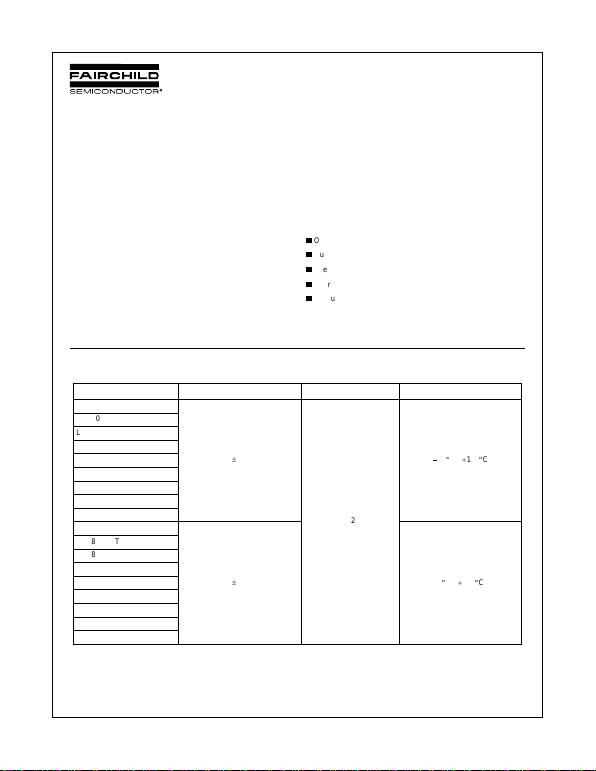© 2005 Fairchild Semiconductor Corporation DS400018 www.fairchildsemi.com
April 1999
Revised December 2005
LM7805 • LM7806 • LM7808 • LM7809 • LM7810 • LM7812 • LM7815 • LM7818 • LM7824 • LM7805A • LM7806A • LM7808A
•LM780 9A • LM7810A • LM7812A • LM7815A • LM7818A • LM7824A 3-Terminal 1A Positive Voltage Regulator
LM7805 • LM7806 • LM7808 • LM7809 •
LM7810 • LM7812 • LM7815 • LM7818 • LM7824 •
LM7805A • LM7806A • LM7808A •LM7809A •
LM7810A • LM7812A • LM7815A • LM7818A • LM7824A
3-Terminal 1A Positi ve Voltage Regulator
General Description
The LM78XX series of three terminal positive regulators are
available in the TO-220 package and with several fixed output
voltages, making them useful in a wide range of applications.
Each type employs internal current limiting, thermal shut down
and safe operating area protection, making it essentially inde-
structible. If adequate heat sinking is provided, they can deliver
over 1A output current. Although designed primarily as fixed
voltage regulators, these devices can be used with external
components to obtain adjustable voltages and currents.
Features
O
Output Current up to 1A
O
Output Voltages of 5, 6, 8, 9, 12, 15, 18, 24
O
O
Short Circuit Protection
O
Output T ransistor Safe Operating Area Protection
Ordering Code:
Product Number Output Voltage Tolerance Package Operating Temperature
LM7805CT
r
4%
TO-220
40
q
C -
125
q
C
LM7806CT
LM7808CT
LM7809CT
LM7810CT
LM7812CT
LM7815CT
LM7818CT
LM7824CT
LM7805ACT
r
2% 0
q
C -
125
q
C
LM7806ACT
LM7808ACT
LM7809ACT
LM7810ACT
LM7812ACT
LM7815ACT
LM7818ACT
LM7824ACT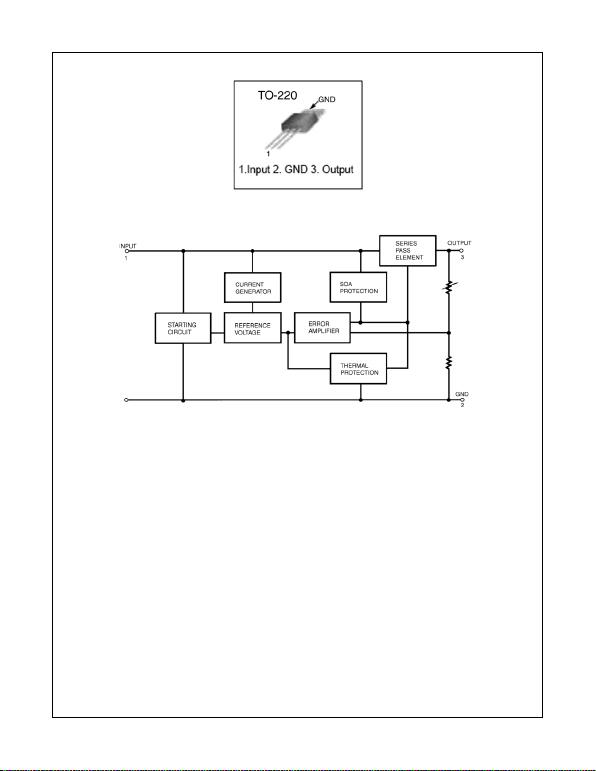www.fairchildsemi.com 2
LM7805 • LM78 06 • LM7808 • LM7809 • LM7810 • LM7812 LM7815 • LM7818 • LM7824 • LM7805A • LM780 6A • LM7808A
•LM7809A • LM7810A • LM7812A • LM7815A • LM7818A • LM7824A
Internal Block Diagram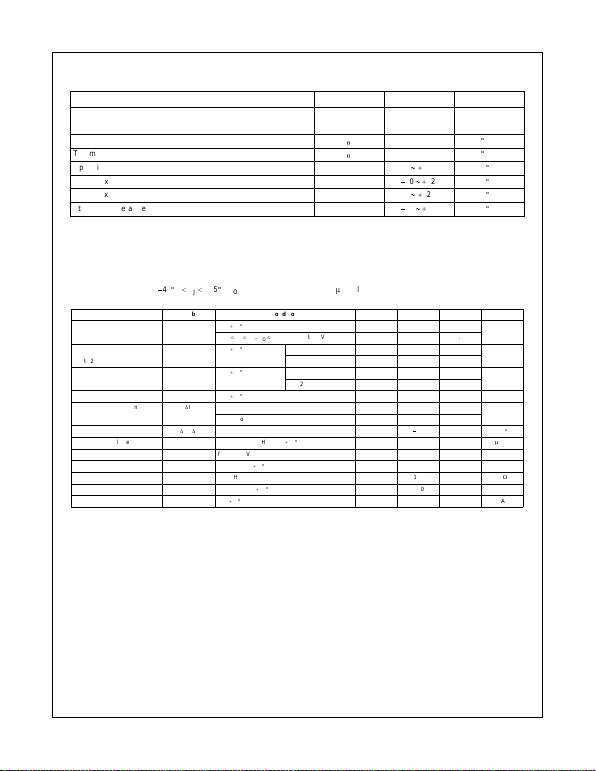3www.fairchildsemi.com
LM7805 • LM7806 • LM7808 • LM7809 • LM7810 • LM7812 • LM7815 • LM7818 • LM7824 • LM7805A • LM7806A • LM7808A
•LM780 9A • LM7810A • LM7812A • LM7815A • LM7818A • LM7824A
Absolute Maximum Ratings(Note 1)
Note 1: Absolute maximum ratings are those values beyond which damage to the device may occur. The datasheet specifications should be met, without exception, to ensure
that the system design is reliable over its power supply, temperature, and output/input loading variables. Fairchild does not recommend operation outside datasheet specifica-
tions.
Electrical Characteristics (LM7805)
(Refer to the test circuits.
40
q
C
TJ
125
q
C, IO = 500mA, VI = 10V, CI = 0.1
P
F, unless otherwise specified)
Note 2: Load and line regulation are specified at constant junction temperature. Changes in VO due to heating effects must be taken into account separately. Pulse testing with
low duty is used.
Note 3: These parameters, although guaranteed, are not 100% tested in production.
Parameter Symbol Value Unit
Input Voltage (for VO = 5V to 18V) VI35 V
(for VO = 24V) VI40 V
Thermal Resistance Junction-Cases (TO-220) R
T
JC 5
q
C/W
Thermal Resistance Junction-Air (TO-220) R
T
JA 65
q
C/W
Operating Temperature Range TOPR 0
a
125
q
C
LM78xx
40
a
125
q
C
LM78xxA 0
a
125
q
C
Storage Temperature Range TSTG
65
a
150
q
C
Parameter Symbol Conditions Min Typ Max Unit
Output Voltage VOTJ =
25
q
C4.85.05.2
V
5mA
d
IO
d
1A, PO
d
15W, VI = 7V to 20V 4.75 5.0 5.25
Line Regulation Regline TJ =
25
q
CV
O = 7V to 25V 4.0 100 mV
(Note 2) VI = 8V to 12V 1.6 50.0
25
q
CI
O = 5mA to 1.5mA 9.0 100 mV
IO = 250mA to 750mA 4.0 50.0
Quiescent Current IQTJ =
25
q
C–5.08.0mA
Quiescent Current Change
'
IQIO = 5mA to 1A 0.03 0.5 mA
VI = 7V to 25V 0.3 1.3
Output Voltage Drift (Note 3)
'
VO/
'
TI
O = 5mA
0.8 mV/
q
C
Output Noise Voltage VNf = 10Hz to 100KHz, TA =
25
q
C 42.0
P
V/VO
Ripple Rejection (Note 3) RR f = 120Hz, VO = 8V to 18V 62.0 73.0 dB
Dropout Voltage VDROP IO = 1A, TJ =
25
q
C–2.0V
Output Resistance (Note 3) rO f = 1KHz 15.0 m
:
Short Circuit Current ISC VI = 35V, TA =
25
q
C–230mA
Peak Current (Note 3) IPK TJ =
25
q
C–2.2A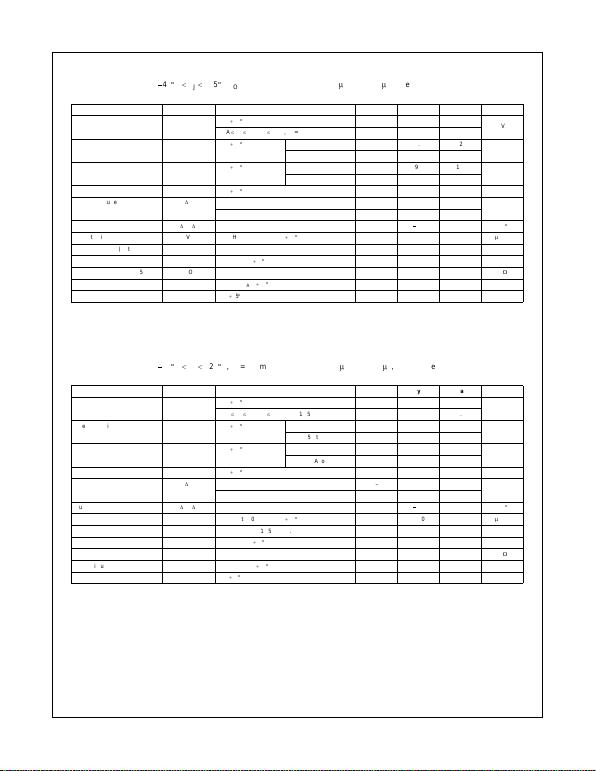www.fairchildsemi.com 4
LM7805 • LM78 06 • LM7808 • LM7809 • LM7810 • LM7812 LM7815 • LM7818 • LM7824 • LM7805A • LM780 6A • LM7808A
•LM7809A • LM7810A • LM7812A • LM7815A • LM7818A • LM7824A
Electrical Characteristics (LM7806)
(Refer to the test circuits.
40
q
C
TJ
125
q
C, IO = 500mA, VI = 11V, CI = 0.33
P
F, CO = 0.1
P
F, unless otherwise specified)
Note 4: Load and line regulation are specified at constant junction temperature. Changes in VO due to heating effects must be taken into account separately. Pulse testing with
low duty is used.
Note 5: These parameters, although guaranteed, are not 100% tested in production.
Electrical Characteristics (LM7808)
(Refer to the test circuits.
40
q
C
TJ
125
q
C, IO = 500mA, VI = 14V, CI = 0.33
P
F, CO = 0.1
P
F, unless otherwise specified)
Note 6: Load and line regulation are specified at constant junction temperature. Changes in VO due to heating effects must be taken into account separately. Pulse testing with
low duty is used.
Note 7: These parameters, although guaranteed, are not 100% tested in production.
Parameter Symbol Conditions Min Typ Max Unit
Output Voltage VOTJ =
25
q
C 5.75 6.0 6.25 V
5mA
d
IO
d
1A, PO
d
15W, VI = 8.0V to 21V 5.7 6.0 6.3
Line Regulation Regline TJ =
25
q
CV
I = 8V to 25V 5.0 120 mV
(Note 4) VI = 9V to 13V 1.5 60.0
25
q
CI
O = 5mA to 1.5mA 9.0 120 mV
(Note 4) IO = 250mA to 750mA 3.0 60.0
Quiescent Current IQTJ =
25
q
C–5.08.0mA
Quiescent Current Change
'
IQIO = 5mA to 1A 0.5 mA
VI = 8V to 25V 1.3
Output Voltage Drift (Note 5)
'
VO/
'
TI
O = 5mA
0.8 mV/
q
C
Output Noise Voltage VNf = 10Hz to 100KHz, TA =
25
q
C 45.0
P
V/VO
Ripple Rejection (Note 5) RR f = 120Hz, VO = 8V to 18V 62.0 73.0 dB
Dropout Voltage VDROP IO = 1A, TJ =
25
q
C–2.0V
Output Resistance (Note 5) rO f = 1KHz 19.0 m
:
Short Circuit Current ISC VI = 35V, TA =
25
q
C–250mA
Peak Current (Note 5) IPK TJ =
25
q
C–2.2A
Parameter Symbol Conditions Min Typ Max Unit
Output Voltage VOTJ =
25
q
C7.78.08.3
V
5mA
d
IO
d
1A, PO
d
15W, VI = 10.5V to 23V 7.6 8.0 8.4
Line Regulation Regline TJ =
25
q
CV
I = 10.5V to 25V 5.0 160 mV
(Note 6) VI = 11.5V to 17V 2.0 80.0
25
q
CI
O = 5mA to 1.5mA 10.0 160 mV
(Note 6) IO = 250mA to 750mA 5.0 80.0
Quiescent Current IQTJ =
25
q
C–5.08.0mA
Quiescent Current Change
'
IQIO = 5mA to 1A 0.05 0.5 mA
VI = 10.5V to 25V 0.5 1.0
Output Voltage Drift (Note 7)
'
VO/
'
TI
O = 5mA
0.8 mV/
q
C
Output Noise Voltage VNf = 10Hz to 100KHz, TA =
25
q
C 52.0
P
V/VO
Ripple Rejection (Note 7) RR f = 120Hz, VO = 11.5V to 21.5V 56.0 73.0 dB
Dropout Voltage VDROP IO = 1A, TJ =
25
q
C–2.0V
Output Resistance (Note 7) rO f = 1KHz 17.0 m
:
Short Circuit Current ISC VI = 35V, TA =
25
q
C–230mA
Peak Current (Note 7) IPK TJ =
25
q
C–2.2A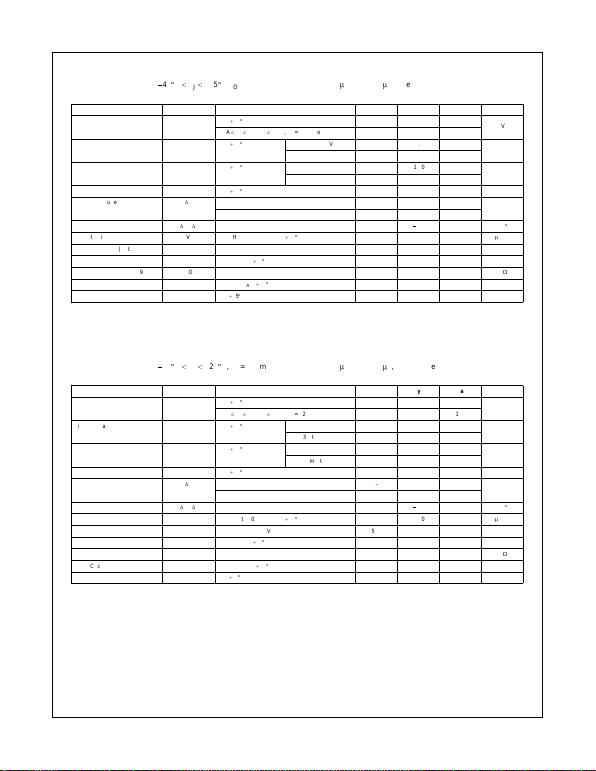5www.fairchildsemi.com
LM7805 • LM7806 • LM7808 • LM7809 • LM7810 • LM7812 • LM7815 • LM7818 • LM7824 • LM7805A • LM7806A • LM7808A
•LM780 9A • LM7810A • LM7812A • LM7815A • LM7818A • LM7824A
Electrical Characteristics (LM7809)
(Refer to the test circuits.
40
q
C
TJ
125
q
C, IO = 500mA, VI = 15V, CI = 0.33
P
F, CO = 0.1
P
F, unless otherwise specified)
Note 8: Load and line regulation are specified at constant junction temperature. Changes in VO due to heating effects must be taken into account separately. Pulse testing with
low duty is used.
Note 9: These parameters, although guaranteed, are not 100% tested in production.
Electrical Characteristics (LM7810)
(Refer to the test circuits.
40
q
C
TJ
125
q
C, IO = 500mA, VI = 16V, CI = 0.33
P
F, CO = 0.1
P
F, unless otherwise specified)
Note 10: Load and line regulation are specified at constant junction temperature. Changes in VO due to heating effects must be taken into account separate ly. Pulse testing with
low duty is used.
Note 11: These parameters, although guaranteed, are not 100% tested in production.
Parameter Symbol Conditions Min Typ Max Unit
Output Voltage VOTJ =
25
q
C 8.65 9.0 9.35 V
5mA
d
IO
d
1A, PO
d
15W, VI = 11.5V to 24V 8.6 9.0 9.4
Line Regulation Regline TJ =
25
q
CV
I = 11.5V to 25V 6.0 180 mV
(Note 8) VI = 12V to 17V 2.0 90.0
25
q
CI
O = 5mA to 1.5mA 12.0 180 mV
(Note 8) IO = 250mA to 750mA 4.0 90.0
Quiescent Current IQTJ =
25
q
C–5.08.0mA
Quiescent Current Change
'
IQIO = 5mA to 1A 0.5 mA
VI = 11.5V to 26V 1.3
Output Voltage Drift (Note 9)
'
VO/
'
TI
O = 5mA
1.0 mV/
q
C
Output Noise Voltage VNf = 10Hz to 100KHz, TA =
25
q
C 58.0
P
V/VO
Ripple Rejection (Note 9) RR f = 120Hz, VO = 13V to 23V 56.0 71.0 dB
Dropout Voltage VDROP IO = 1A, TJ =
25
q
C–2.0V
Output Resistance (Note 9) rO f = 1KHz 17.0 m
:
Short Circuit Current ISC VI = 35V, TA =
25
q
C–250mA
Peak Current (Note 9) IPK TJ =
25
q
C–2.2A
Parameter Symbol Conditions Min Typ Max Unit
Output Voltage VOTJ =
25
q
C 9.6 10.0 10.4 V
5mA
d
IO
d
1A, PO
d
15W, VI = 12.5V to 25V 9.5 10.0 10.5
Line Regulation Regline TJ =
25
q
CV
I = 12.5V to 25V 10.0 200 mV
(Note 10) VI = 13V to 25V 3.0 100
25
q
CI
O = 5mA to 1.5mA 12.0 200 mV
(Note 10) IO = 250mA to 750mA 4.0 400
Quiescent Current IQTJ =
25
q
C–5.18.0mA
Quiescent Current Change
'
IQIO = 5mA to 1A 0.5 mA
VI = 12.5V to 29V 1.0
Output Voltage Drift (Note 11)
'
VO/
'
TI
O = 5mA
1.0 mV/
q
C
Output Noise Voltage VNf = 10Hz to 100KHz, TA =
25
q
C 58.0
P
V/VO
Ripple Rejection (Note 11) RR f = 120Hz, VO = 13V to 23V 56.0 71.0 dB
Dropout Voltage VDROP IO = 1A, TJ =
25
q
C–2.0V
Output Resistance (Note 11) rO f = 1KHz 17.0 m
:
Short Circuit Current ISC VI = 35V, TA =
25
q
C–250mA
Peak Current (Note 11) IPK TJ =
25
q
C–2.2A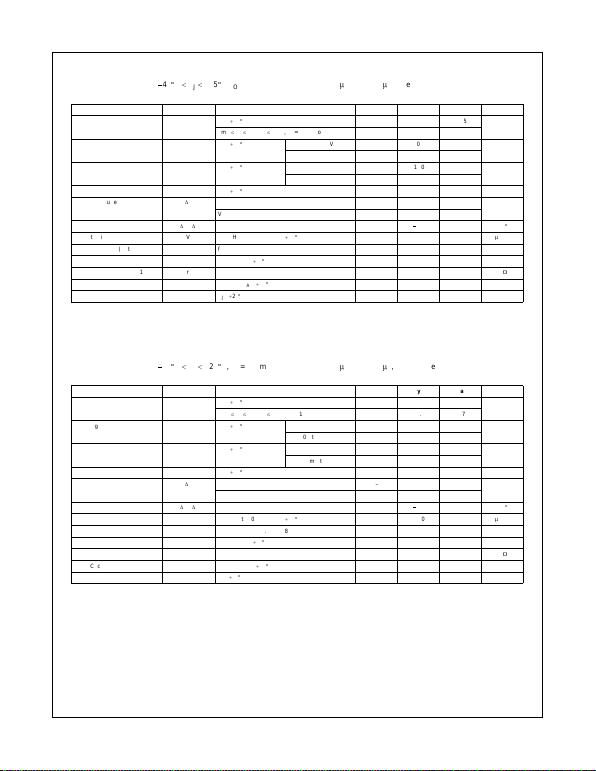www.fairchildsemi.com 6
LM7805 • LM78 06 • LM7808 • LM7809 • LM7810 • LM7812 LM7815 • LM7818 • LM7824 • LM7805A • LM780 6A • LM7808A
•LM7809A • LM7810A • LM7812A • LM7815A • LM7818A • LM7824A
Electrical Characteristics (LM7812)
(Refer to the test circuits.
40
q
C
TJ
125
q
C, IO = 500mA, VI = 19V, CI = 0.33
P
F, CO = 0.1
P
F, unless otherwise specified)
Note 12: Load and line regulation are specified at constant junction temperature. Changes in VO due to heating effects must be taken into account separate ly. Pulse testing with
low duty is used.
Note 13: These parameters, although guaranteed, are not 100% tested in production.
Electrical Characteristics (LM7815)
(Refer to the test circuits.
40
q
C
TJ
125
q
C, IO = 500mA, VI = 23V, CI = 0.33
P
F, CO = 0.1
P
F, unless otherwise specified)
Note 14: Load and line regulation are specified at constant junction temperature. Changes in VO due to heating effects must be taken into account separate ly. Pulse testing with
low duty is used.
Note 15: These parameters, although guaranteed, are not 100% tested in production.
Parameter Symbol Conditions Min Typ Max Unit
Output Voltage VOTJ =
25
q
C 11.5 12.0 12.5 V
5mA
d
IO
d
1A, PO
d
15W, VI = 14.5V to 27V 11.4 12.0 12.6
Line Regulation Regline TJ =
25
q
CV
I = 14.5V to 30V 10.0 240 mV
(Note 12) VI = 16V to 22V 3.0 120
25
q
CI
O = 5mA to 1.5mA 11.0 240 mV
(Note 12) IO = 250mA to 750mA 5.0 120
Quiescent Current IQTJ =
25
q
C–5.18.0mA
Quiescent Current Change
'
IQIO = 5mA to 1A 0.1 0.5 mA
VI = 14.5V to 30V 0.5 1.0
Output Voltage Drift (Note 13)
'
VO/
'
TI
O = 5mA
1.0 mV/
q
C
Output Noise Voltage VNf = 10Hz to 100KHz, TA =
25
q
C 76.0
P
V/VO
Ripple Rejection (Note 13) RR f = 120Hz, VI = 15V to 25V 55.0 71.0 dB
Dropout Voltage VDROP IO = 1A, TJ =
25
q
C–2.0V
Output Resistance (Note 13) rO f = 1KHz 18.0 m
:
Short Circuit Current ISC VI = 35V, TA =
25
q
C–230mA
Peak Current (Note 13) IPK TJ =
25
q
C–2.2A
Parameter Symbol Conditions Min Typ Max Unit
Output Voltage VOTJ =
25
q
C 14.4 15.0 15.6 V
5mA
d
IO
d
1A, PO
d
15W, VI = 17.5V to 30V 14.25 15.0 15.75
Line Regulation Regline TJ =
25
q
CV
I = 17.5V to 30V 11.0 300 mV
(Note 14) VI = 20V to 26V 3.0 150
25
q
CI
O = 5mA to 1.5mA 12.0 300 mV
(Note 14) IO = 250mA to 750mA 4.0 150
Quiescent Current IQTJ =
25
q
C–5.28.0mA
Quiescent Current Change
'
IQIO = 5mA to 1A 0.5 mA
VI = 17.5V to 30V 1.0
Output Voltage Drift (Note 15)
'
VO/
'
TI
O = 5mA
1.0 mV/
q
C
Output Noise Voltage VNf = 10Hz to 100KHz, TA =
25
q
C 90.0
P
V/VO
Ripple Rejection (Note 15) RR f = 120Hz, VI = 18.5V to 28.5V 54.0 70.0 dB
Dropout Voltage VDROP IO = 1A, TJ =
25
q
C–2.0V
Output Resistance (Note 15) rO f = 1KHz 19.0 m
:
Short Circuit Current ISC VI = 35V, TA =
25
q
C–250mA
Peak Current (Note 15) IPK TJ =
25
q
C–2.2A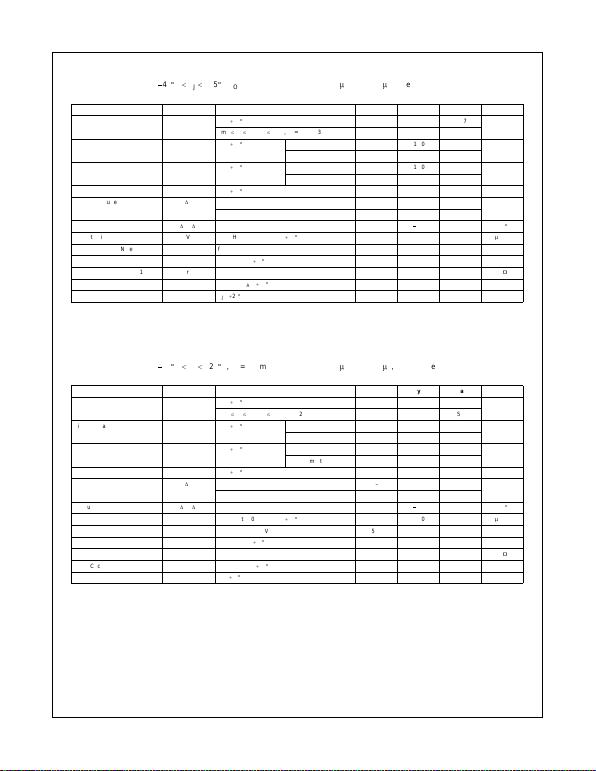7www.fairchildsemi.com
LM7805 • LM7806 • LM7808 • LM7809 • LM7810 • LM7812 • LM7815 • LM7818 • LM7824 • LM7805A • LM7806A • LM7808A
•LM780 9A • LM7810A • LM7812A • LM7815A • LM7818A • LM7824A
Electrical Characteristics (LM7818)
(Refer to the test circuits.
40
q
C
TJ
125
q
C, IO = 500mA, VI = 27V, CI = 0.33
P
F, CO = 0.1
P
F, unless otherwise specified)
Note 16: Load and line regulation are specified at constant junction temperature. Changes in VO due to heating effects must be taken into account separate ly. Pulse testing with
low duty is used.
Note 17: These parameters, although guaranteed, are not 100% tested in production.
Electrical Characteristics (LM7824)
(Refer to the test circuits.
40
q
C
TJ
125
q
C, IO = 500mA, VI = 33V, CI = 0.33
P
F, CO = 0.1
P
F, unless otherwise specified)
Note 18: Load and line regulation are specified at constant junction temperature. Changes in VO due to heating effects must be taken into account separate ly. Pulse testing with
low duty is used.
Note 19: These parameters, although guaranteed, are not 100% tested in production.
Parameter Symbol Conditions Min Typ Max Unit
Output Voltage VOTJ =
25
q
C 17.3 18.0 18.7 V
5mA
d
IO
d
1A, PO
d
15W, VI = 21V to 33V 17.1 18.0 18.9
Line Regulation Regline TJ =
25
q
CV
I = 21V to 33V 15.0 360 mV
(Note 12) VI = 24V to 30V 5.0 180
25
q
CI
O = 5mA to 1.5mA 15.0 360 mV
(Note 12) IO = 250mA to 750mA 5.0 180
Quiescent Current IQTJ =
25
q
C–5.28.0mA
Quiescent Current Change
'
IQIO = 5mA to 1A 0.5 mA
VI = 21V to 33V 1.0
Output Voltage Drift (Note 17)
'
VO/
'
TI
O = 5mA
1.0 mV/
q
C
Output Noise Voltage VNf = 10Hz to 100KHz, TA =
25
q
C–110
P
V/VO
Ripple Rejection (Note 17) RR f = 120Hz, VI = 22V to 32V 53.0 69.0 dB
Dropout Voltage VDROP IO = 1A, TJ =
25
q
C–2.0V
Output Resistance (Note 17) rO f = 1KHz 22.0 m
:
Short Circuit Current ISC VI = 35V, TA =
25
q
C–250mA
Peak Current (Note 17) IPK TJ =
25
q
C–2.2A
Parameter Symbol Conditions Min Typ Max Unit
Output Voltage VOTJ =
25
q
C 23.0 24.0 25.0 V
5mA
d
IO
d
1A, PO
d
15W, VI = 27V to 38V 22.8 24.0 25.25
Line Regulation Regline TJ =
25
q
CV
I = 27V to 38V 17.0 480 mV
(Note 18) VI = 30V to 36V 6.0 240
25
q
CI
O = 5mA to 1.5mA 15.0 480 mV
(Note 18) IO = 250mA to 750mA 5.0 240
Quiescent Current IQTJ =
25
q
C–5.28.0mA
Quiescent Current Change
'
IQIO = 5mA to 1A 0.1 0.5 mA
VI = 27V to 38V 0.5 1.0
Output Voltage Drift (Note 19)
'
VO/
'
TI
O = 5mA
1.5 mV/
q
C
Output Noise Voltage VNf = 10Hz to 100KHz, TA =
25
q
C 60.0
P
V/VO
Ripple Rejection (Note 19) RR f = 120Hz, VI = 28V to 38V 50.0 67.0 dB
Dropout Voltage VDROP IO = 1A, TJ =
25
q
C–2.0V
Output Resistance (Note 19) rO f = 1KHz 28.0 m
:
Short Circuit Current ISC VI = 35V, TA =
25
q
C–230mA
Peak Current (Note 19) IPK TJ =
25
q
C–2.2A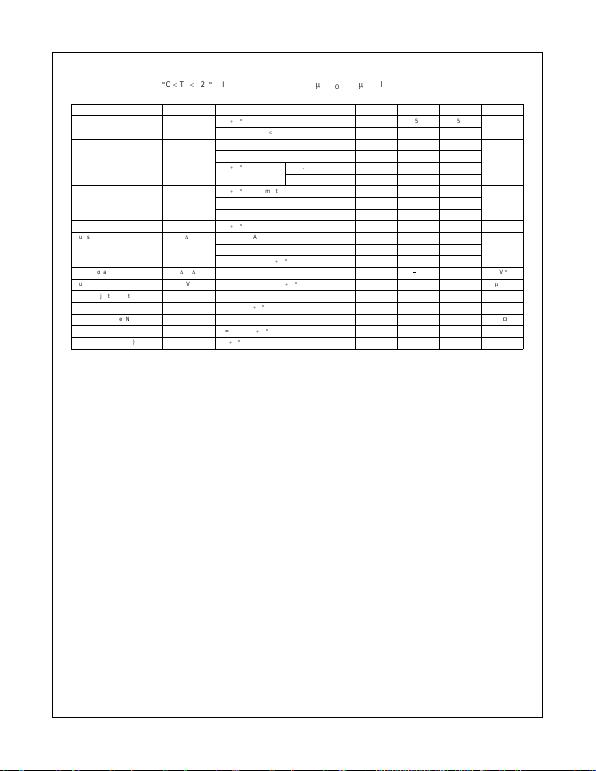www.fairchildsemi.com 8
LM7805 • LM78 06 • LM7808 • LM7809 • LM7810 • LM7812 LM7815 • LM7818 • LM7824 • LM7805A • LM780 6A • LM7808A
•LM7809A • LM7810A • LM7812A • LM7815A • LM7818A • LM7824A
Electrical Characteristics (LM7805A)
(Refer to the test circuits. 0
q
C
TJ
125
q
C, IO = 1A, VI = 10V, CI = 0.33
P
F, CO = 0.1
P
F, unless otherwise specified)
Note 20: Load and line regulation are specified at constant junction temperature. Changes in VO due to heating effects must be taken into account separate ly. Pulse testing with
low duty is used.
Note 21: These parameters, although guaranteed, are not 100% tested in production.
Parameter Symbol Conditions Min Typ Max Unit
Output Voltage VOTJ =
25
q
C4.95.05.1
V
IO = 5mA to 1A, PO
d
15W, VI = 7.5V to 20V 4.8 5.0‘ 5.2
Line Regulation Regline VI = 7.5V to 25V, IO = 500mA 5.0 50.0
mV
(Note 20) VI = 8V to 12V 3.0 50.0
TJ =
25
q
CV
I = 7.3V to 20V 5.0 50.0
VI = 8V to 12V 1.5 25.0
25
q
C, IO = 5mA to 1.5mA 9.0 100
mV(Note 20) IO = 5mA to 1mA 9.0 100
IO = 250mA to 750mA 4.0 50.0
Quiescent Current IQTJ =
25
q
C–5.06.0mA
Quiescent Current Change
'
IQIO = 5mA to 1A 0.5
mAVI = 8V to 25V, IO = 500mA 0.8
VI = 7.5V to 20V, TJ =
25
q
C–0.8
Output Voltage Drift (Note 21)
'
VO/
'
TI
O = 5mA
0.8 mV/
q
C
Output Noise Voltage VNf = 10Hz to 100KHz, TA =
25
q
C 10.0
P
V/VO
Ripple Rejection (Note 21) RR f = 120Hz, IO = 500mA, VI = 8V to 18V 68.0 dB
Dropout Voltage VDROP IO = 1A, TJ =
25
q
C–2.0V
Output Resistance (Note 21) rO f = 1KHz 17.0 m
:
Short Circuit Current ISC VI = 35V, TA =
25
q
C–250mA
Peak Current (Note 21) IPK TJ =
25
q
C–2.2A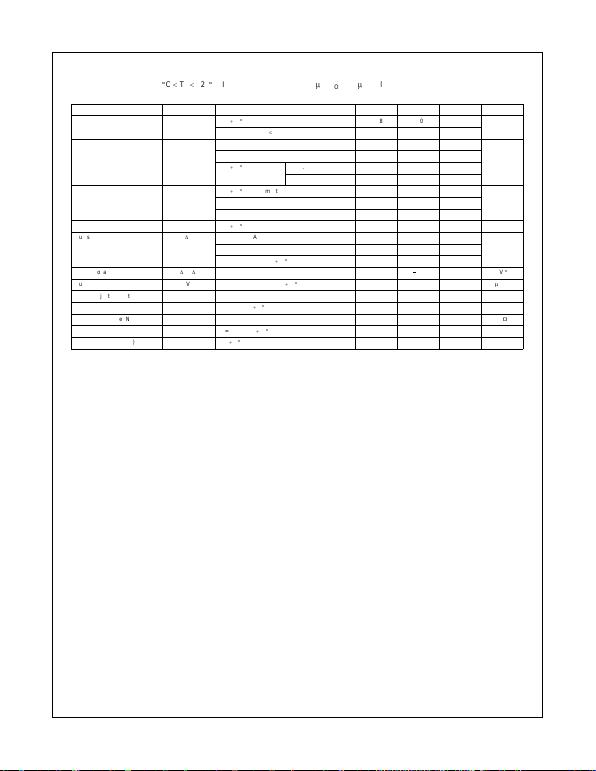9www.fairchildsemi.com
LM7805 • LM7806 • LM7808 • LM7809 • LM7810 • LM7812 • LM7815 • LM7818 • LM7824 • LM7805A • LM7806A • LM7808A
•LM780 9A • LM7810A • LM7812A • LM7815A • LM7818A • LM7824A
Electrical Characteristics (LM7806A)
(Refer to the test circuits. 0
q
C
TJ
125
q
C, IO = 1A, VI = 11V, CI = 0.33
P
F, CO = 0.1
P
F, unless otherwise specified)
Note 22: Load and line regulation are specified at constant junction temperature. Changes in VO due to heating effects must be taken into account separate ly. Pulse testing with
low duty is used.
Note 23: These parameters, although guaranteed, are not 100% tested in production.
Parameter Symbol Conditions Min Typ Max Unit
Output Voltage VOTJ =
25
q
C 5.58 6.0 6.12 V
IO = 5mA to 1A, PO
d
15W, VI = 8.6V to 21V 5.76 6.0 6.24
Line Regulation Regline VI = 8.6V to 25V, IO = 500mA 5.0 60.0
mV
(Note 22) VI = 9V to 13V 3.0 60.0
TJ =
25
q
CV
I = 8.3V to 21V 5.0 60.0
VI = 9V to 13V 1.5 30.0
25
q
C, IO = 5mA to 1.5mA 9.0 100
mV(Note 22) IO = 5mA to 1mA 4.0 100
IO = 250mA to 750mA 5.0 50.0
Quiescent Current IQTJ =
25
q
C–4.36.0mA
Quiescent Current Change
'
IQIO = 5mA to 1A 0.5
mAVI = 19V to 25V, IO = 500mA 0.8
VI = 8.5V to 21V, TJ =
25
q
C–0.8
Output Voltage Drift (Note 23)
'
VO/
'
TI
O = 5mA
0.8 mV/
q
C
Output Noise Voltage VNf = 10Hz to 100KHz, TA =
25
q
C 10.0
P
V/VO
Ripple Rejection (Note 23) RR f = 120Hz, IO = 500mA, VI = 9V to 19V 65.0 dB
Dropout Voltage VDROP IO = 1A, TJ =
25
q
C–2.0V
Output Resistance (Note 23) rO f = 1KHz 17.0 m
:
Short Circuit Current ISC VI = 35V, TA =
25
q
C–250mA
Peak Current (Note 23) IPK TJ =
25
q
C–2.2A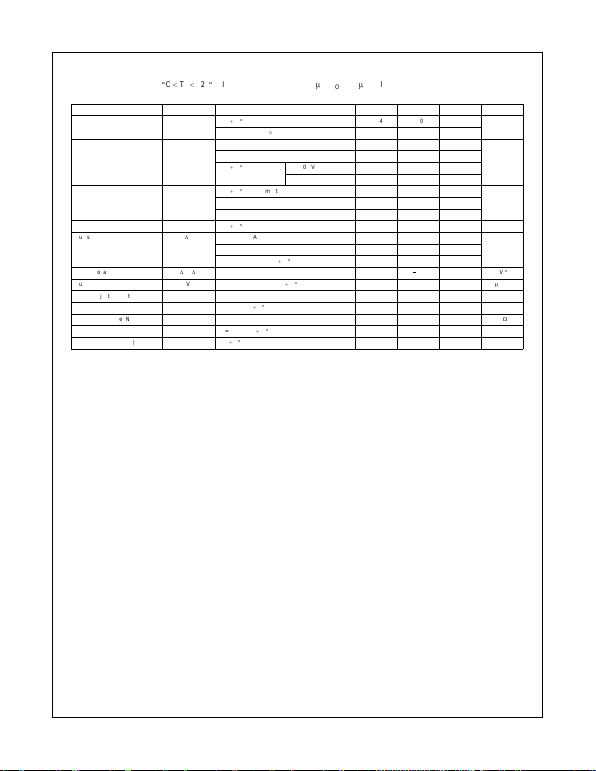www.fairchildsemi.com 10
LM7805 • LM78 06 • LM7808 • LM7809 • LM7810 • LM7812 LM7815 • LM7818 • LM7824 • LM7805A • LM780 6A • LM7808A
•LM7809A • LM7810A • LM7812A • LM7815A • LM7818A • LM7824A
Electrical Characteristics (LM7808A)
(Refer to the test circuits. 0
q
C
TJ
125
q
C, IO = 1A, VI = 14V, CI = 0.33
P
F, CO = 0.1
P
F, unless otherwise specified)
Note 24: Load and line regulation are specified at constant junction temperature. Changes in VO due to heating effects must be taken into account separate ly. Pulse testing with
low duty is used.
Note 25: These parameters, although guaranteed, are not 100% tested in production.
Parameter Symbol Conditions Min Typ Max Unit
Output Voltage VOTJ =
25
q
C 7.84 8.0 8.16 V
IO = 5mA to 1A, PO
d
15W, VI = 10.6V to 23V 7.7 8.0 8.3
Line Regulation Regline VI = 10.6V to 25V, IO = 500mA 6.0 80.0
mV
(Note 24) VI = 11V to 17V 3.0 80.0
TJ =
25
q
CV
I = 10.4V to 23V 6.0 80.0
VI = 11V to 17V 2.0 40.0
25
q
C, IO = 5mA to 1.5mA 12.0 100
mV(Note 24) IO = 5mA to 1mA 12.0 100
IO = 250mA to 750mA 5.0 50.0
Quiescent Current IQTJ =
25
q
C–5.06.0mA
Quiescent Current Change
'
IQIO = 5mA to 1A 0.5
mAVI = 11V to 25V, IO = 500mA 0.8
VI = 10.6V to 23V, TJ =
25
q
C–0.8
Output Voltage Drift (Note 25)
'
VO/
'
TI
O = 5mA
0.8 mV/
q
C
Output Noise Voltage VNf = 10Hz to 100KHz, TA =
25
q
C 10.0
P
V/VO
Ripple Rejection (Note 25) RR f = 120Hz, IO = 500mA, VI = 11.5V to 21.5V 62.0 dB
Dropout Voltage VDROP IO = 1A, TJ =
25
q
C–2.0V
Output Resistance (Note 25) rO f = 1KHz 18.0 m
:
Short Circuit Current ISC VI = 35V, TA =
25
q
C–250mA
Peak Current (Note 25) IPK TJ =
25
q
C–2.2A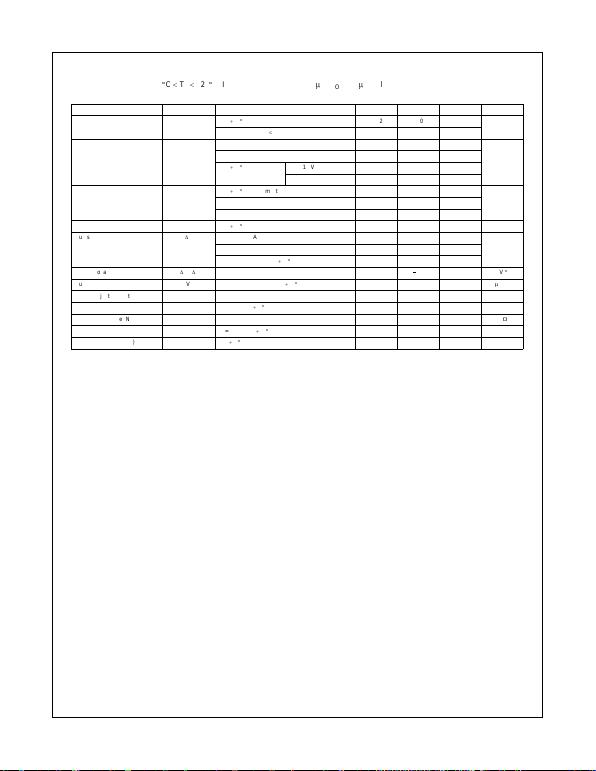11 www.fairchildsemi.com
LM7805 • LM7806 • LM7808 • LM7809 • LM7810 • LM7812 • LM7815 • LM7818 • LM7824 • LM7805A • LM7806A • LM7808A
•LM780 9A • LM7810A • LM7812A • LM7815A • LM7818A • LM7824A
Electrical Characteristics (LM7809A)
(Refer to the test circuits. 0
q
C
TJ
125
q
C, IO = 1A, VI = 15V, CI = 0.33
P
F, CO = 0.1
P
F, unless otherwise specified)
Note 26: Load and line regulation are specified at constant junction temperature. Changes in VO due to heating effects must be taken into account separate ly. Pulse testing with
low duty is used.
Note 27: These parameters, although guaranteed, are not 100% tested in production.
Parameter Symbol Conditions Min Typ Max Units
Output Voltage VOTJ =
25
q
C 8.82 9.0 9.16 V
IO = 5mA to 1A, PO
d
15W, VI = 11.2V to 24V 8.65 9.0 9.35
Line Regulation Regline VI = 11.7V to 25V, IO = 500mA 6.0 90.0
mV
(Note 26) VI = 12.5V to 19V 4.0 45.0
TJ =
25
q
CV
I = 11.5V to 24V 6.0 90.0
VI = 12.5V to 19V 2.0 45.0
25
q
C, IO = 5mA to 1.0mA 12.0 100
mV(Note 26) IO = 5mA to 1mA 12.0 100
IO = 250mA to 750mA 5.0 50.0
Quiescent Current IQTJ =
25
q
C–5.06.0mA
Quiescent Current Change
'
IQIO = 5mA to 1A 0.5
mAVI = 12V to 25V, IO = 500mA 0.8
VI = 11.7V to 25V, TJ =
25
q
C–0.8
Output Voltage Drift (Note 27)
'
VO/
'
TI
O = 5mA
1.0 mV/
q
C
Output Noise Voltage VNf = 10Hz to 100KHz, TA =
25
q
C 10.0
P
V/VO
Ripple Rejection (Note 27) RR f = 120Hz, IO = 500mA, VI = 12V to 22V 62.0 dB
Dropout Voltage VDROP IO = 1A, TJ =
25
q
C–2.0V
Output Resistance (Note 27) rO f = 1KHz 17.0 m
:
Short Circuit Current ISC VI = 35V, TA =
25
q
C–250mA
Peak Current (Note 27) IPK TJ =
25
q
C–2.2A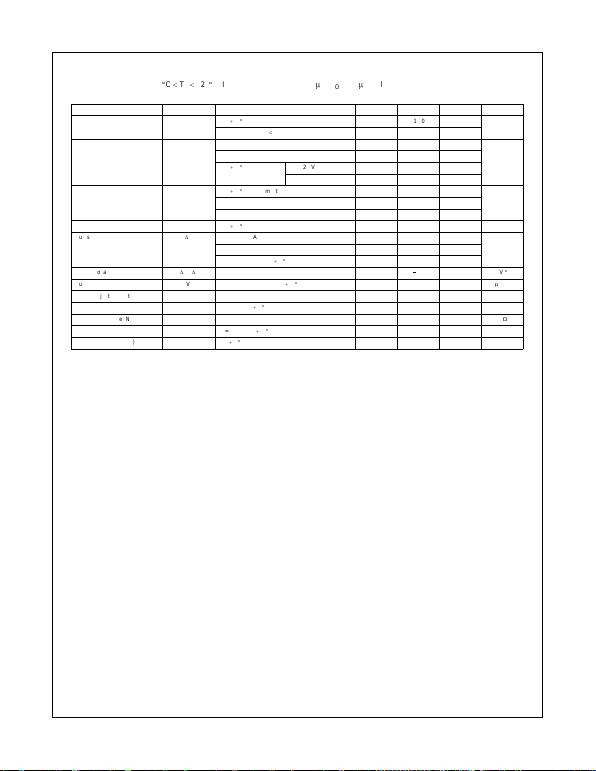www.fairchildsemi.com 12
LM7805 • LM78 06 • LM7808 • LM7809 • LM7810 • LM7812 LM7815 • LM7818 • LM7824 • LM7805A • LM780 6A • LM7808A
•LM7809A • LM7810A • LM7812A • LM7815A • LM7818A • LM7824A
Electrical Characteristics (LM7810A)
(Refer to the test circuits. 0
q
C
TJ
125
q
C, IO = 1A, VI = 16V, CI = 0.33
P
F, CO = 0.1
P
F, unless otherwise specified)
Note 28: Load and line regulation are specified at constant junction temperature. Changes in VO due to heating effects must be taken into account separate ly. Pulse testing with
low duty is used.
Note 29: These parameters, although guaranteed, are not 100% tested in production.
Parameter Symbol Conditions Min Typ Max Units
Output Voltage VOTJ =
25
q
C 9.8 10.0 10.2 V
IO = 5mA to 1A, PO
d
15W, VI = 12.8V to 25V 9.6 10.0 10.4
Line Regulation Regline VI = 12.8V to 26V, IO = 500mA 8.0 100
mV
(Note 28) VI = 13V to 20V 4.0 50.0
TJ =
25
q
CV
I = 12.5V to 25V 8.0 100
VI = 13V to 20V 3.0 50.0
25
q
C, IO = 5mA to 1.5mA 12.0 100
mV(Note 28) IO = 5mA to 1mA 12.0 100
IO = 250mA to 750mA 5.0 50.0
Quiescent Current IQTJ =
25
q
C–5.06.0mA
Quiescent Current Change
'
IQIO = 5mA to 1A 0.5
mAVI = 12.8V to 25V, IO = 500mA 0.8
VI = 13V to 26V, TJ =
25
q
C–0.5
Output Voltage Drift (Note 29)
'
VO/
'
TI
O = 5mA
1.0 mV/
q
C
Output Noise Voltage VNf = 10Hz to 100KHz, TA =
25
q
C 10.0
P
V/VO
Ripple Rejection (Note 29) RR f = 120Hz, IO = 500mA, VI = 14V to 24V 62.0 dB
Dropout Voltage VDROP IO = 1A, TJ =
25
q
C–2.0V
Output Resistance (Note 29) rO f = 1KHz 17.0 m
:
Short Circuit Current ISC VI = 35V, TA =
25
q
C–250mA
Peak Current (Note 29) IPK TJ =
25
q
C–2.2A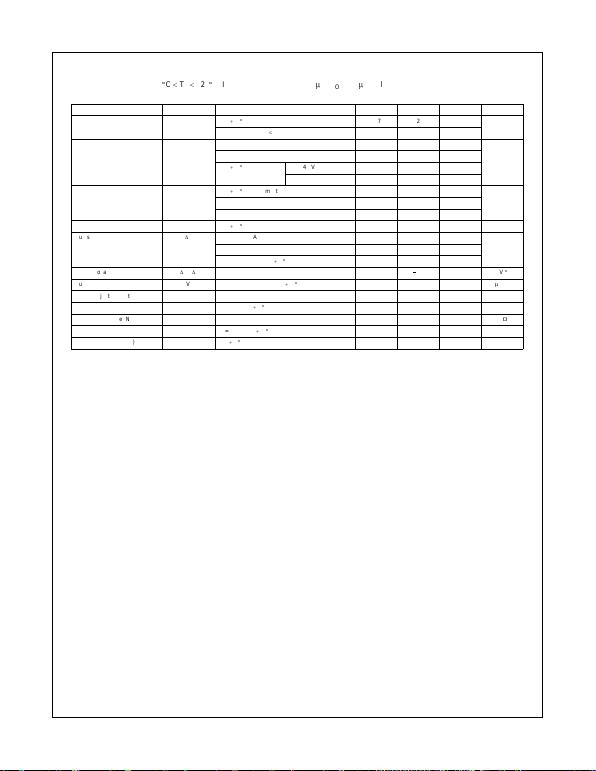13 www.fairchildsemi.com
LM7805 • LM7806 • LM7808 • LM7809 • LM7810 • LM7812 • LM7815 • LM7818 • LM7824 • LM7805A • LM7806A • LM7808A
•LM780 9A • LM7810A • LM7812A • LM7815A • LM7818A • LM7824A
Electrical Characteristics (LM7812A)
(Refer to the test circuits. 0
q
C
TJ
125
q
C, IO = 1A, VI = 19V, CI = 0.33
P
F, CO = 0.1
P
F, unless otherwise specified)
Note 30: Load and line regulation are specified at constant junction temperature. Changes in VO due to heating effects must be taken into account separate ly. Pulse testing with
low duty is used.
Note 31: These parameters, although guaranteed, are not 100% tested in production.
Parameter Symbol Conditions Min Typ Max Units
Output Voltage VOTJ =
25
q
C 11.75 12.0 12.25 V
IO = 5mA to 1A, PO
d
15W, VI = 14.8V to 27V 11.5 12.0 12.5
Line Regulation Regline VI = 14.8V to 30V, IO = 500mA 10.0 120
mV
(Note 30) VI = 16V to 22V 4.0 120
TJ =
25
q
CV
I = 14.5V to 27V 10.0 120
VI = 16V to 22V 3.0 60.0
25
q
C, IO = 5mA to 1.5mA 12.0 100
mV(Note 30) IO = 5mA to 1mA 12.0 100
IO = 250mA to 750mA 5.0 50.0
Quiescent Current IQTJ =
25
q
C–5.16.0mA
Quiescent Current Change
'
IQIO = 5mA to 1A 0.5
mAVI = 14V to 27V, IO = 500mA 0.8
VI = 15V to 30V, TJ =
25
q
C–0.8
Output Voltage Drift (Note 31)
'
VO/
'
TI
O = 5mA
1.0 mV/
q
C
Output Noise Voltage VNf = 10Hz to 100KHz, TA =
25
q
C 10.0
P
V/VO
Ripple Rejection (Note 31) RR f = 120Hz, IO = 500mA, VI = 14V to 24V 60.0 dB
Dropout Voltage VDROP IO = 1A, TJ =
25
q
C–2.0V
Output Resistance (Note 31) rO f = 1KHz 18.0 m
:
Short Circuit Current ISC VI = 35V, TA =
25
q
C–250mA
Peak Current (Note 31) IPK TJ =
25
q
C–2.2A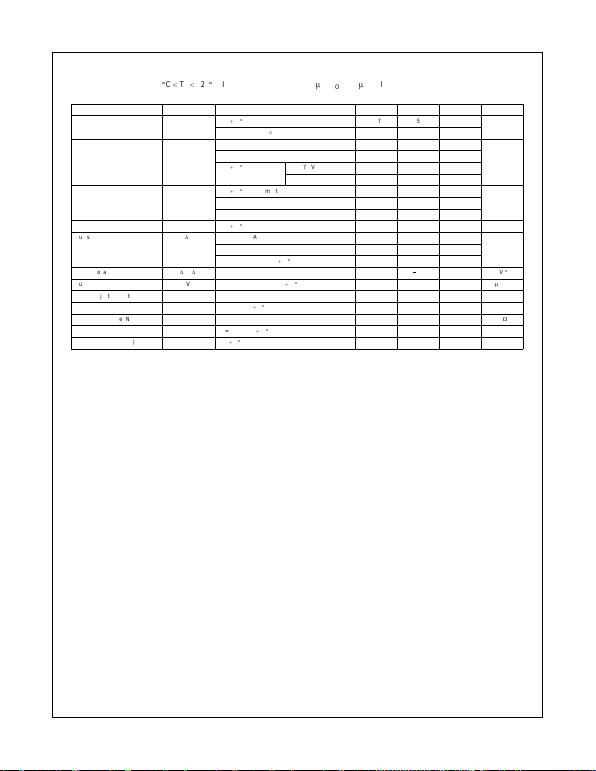www.fairchildsemi.com 14
LM7805 • LM78 06 • LM7808 • LM7809 • LM7810 • LM7812 LM7815 • LM7818 • LM7824 • LM7805A • LM780 6A • LM7808A
•LM7809A • LM7810A • LM7812A • LM7815A • LM7818A • LM7824A
Electrical Characteristics (LM7815A)
(Refer to the test circuits. 0
q
C
TJ
125
q
C, IO = 1A, VI = 23V, CI = 0.33
P
F, CO = 0.1
P
F, unless otherwise specified)
Note 32: Load and line regulation are specified at constant junction temperature. Changes in VO due to heating effects must be taken into account separate ly. Pulse testing with
low duty is used.
Note 33: These parameters, although guaranteed, are not 100% tested in production.
Parameter Symbol Conditions Min Typ Max Units
Output Voltage VOTJ =
25
q
C 14.75 15.0 15.3 V
IO = 5mA to 1A, PO
d
15W, VI = 17.7V to 30V 14.4 15.0 15.6
Line Regulation Regline VI = 17.4V to 30V, IO = 500mA 10.0 150
mV
(Note 32) VI = 20V to 26V 5.0 150
TJ =
25
q
CV
I = 17.5V to 30V 11.0 150
VI = 20V to 26V 3.0 75.0
25
q
C, IO = 5mA to 1.5mA 12.0 100
mV(Note 32) IO = 5mA to 1mA 12.0 100
IO = 250mA to 750mA 5.0 50.0
Quiescent Current IQTJ =
25
q
C–5.26.0mA
Quiescent Current Change
'
IQIO = 5mA to 1A 0.5
mAVI = 17.5V to 30V, IO = 500mA 0.8
VI = 17.5V to 30V, TJ =
25
q
C–0.8
Output Voltage Drift (Note 33)
'
VO/
'
TI
O = 5mA
1.0 mV/
q
C
Output Noise Voltage VNf = 10Hz to 100KHz, TA =
25
q
C 10.0
P
V/VO
Ripple Rejection (Note 33) RR f = 120Hz, IO = 500mA, VI = 18 .5V to 28.5V 58.0 dB
Dropout Voltage VDROP IO = 1A, TJ =
25
q
C–2.0V
Output Resistance (Note 33) rO f = 1KHz 19.0 m
:
Short Circuit Current ISC VI = 35V, TA =
25
q
C–250mA
Peak Current (Note 33) IPK TJ =
25
q
C–2.2A